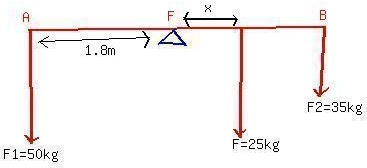# Three Children are trying to balance on a seesaw, which consists of a fulcrum rock, acting as a pivot at the center, and avery light board 3.6 m long.postillan4 2021-02-19 Answered
Three Children are trying to balance on a seesaw, which consists of a fulcrum rock, acting as a pivot at the center, and avery light board 3.6 m long. Two playmates are already on either end. Boy A has a mass of 50kg, and girl B a mass of 35 kg. Where should girl C, whose mass is 25 kg, placed herself so as to balance the seesaw.
You can still ask an expert for help

• Questions are typically answered in as fast as 30 minutes

Solve your problem for the price of one coffee

• Math expert for every subject
• Pay only if we can solve itfaldduETaking the torques acting about the fulcrum F we have, ${F}_{1}\cdot 1.8=F\cdot x+{F}_{2}\cdot 1.8$
Substituting the values of ${F}_{1},{F}_{2}$ and F, the distance 'x' is calculated
Or $x=\frac{\left(50\cdot 1.8\right)-\left(35\cdot 1.8\right)}{25}$
Or x=1.08 m or 1.1 m